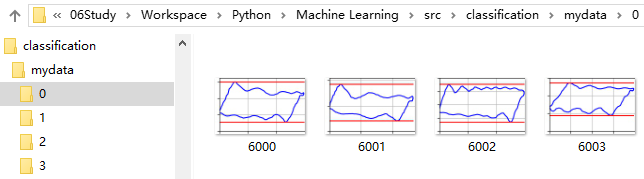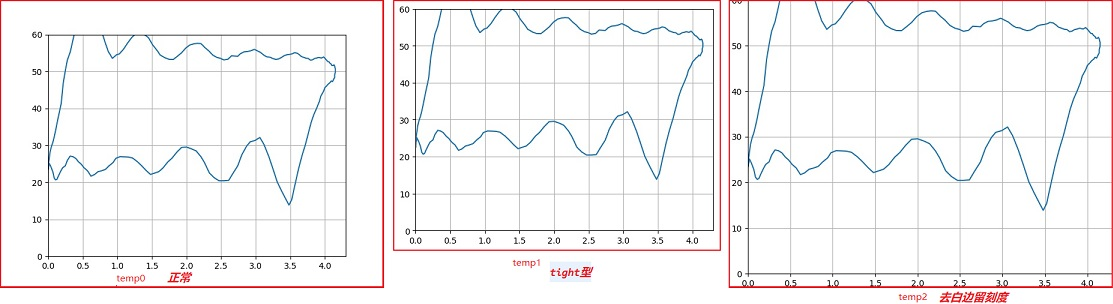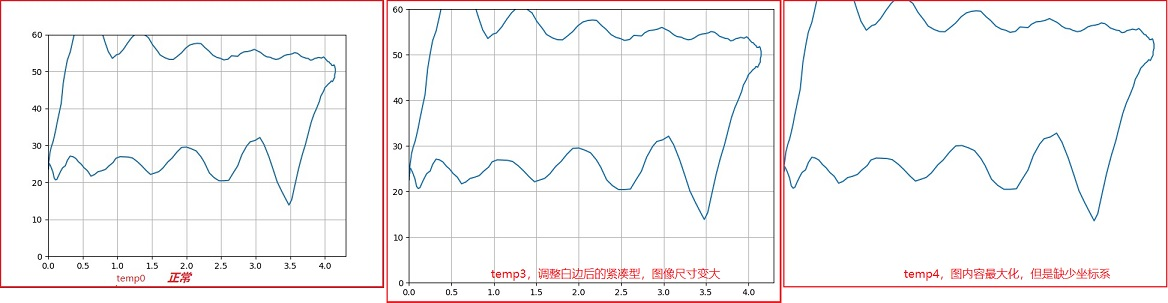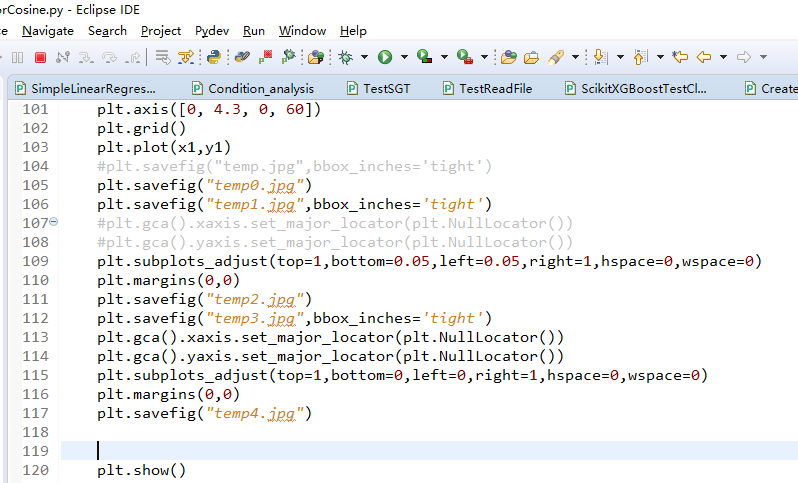# 1. 创建目录def mkdir(path):
# 去除首位空格
path=path.strip()
# 去除尾部 \ 符号
path=path.rstrip("\\")
# 判断路径是否存在，存在——True；不存在——False
isExists=os.path.exists(path)
# 判断结果
if not isExists:
# 如果不存在则创建目录，创建目录操作函数
os.makedirs(path)
return True
else:
# 如果目录存在则不创建，并提示目录已存在
return False


# 3. 绘制图形并保存为图片

## 3.1. 创建画板

plt.figure(figsize(1.92, 1.2)) # 设置 figsize 192*120

## 3.3. 画图

plt.plot(x,y,color="Blue")
plt.plot(upx,upy,color="Red")
plt.plot(downx,downy,color="Red")


## 3.4. 保存图片## 3.5. 关闭画板

while row < c:
tmp_data = data_X[row]
......
#1.创建画板
plt.figure(figsize(1.92, 1.2)) # 设置 figsize 192*120
#plt.rcParams['savefig.dpi'] = 300 #图片像素
#plt.rcParams['figure.dpi'] = 300 #分辨率
# 默认的像素：[6.0,4.0]，分辨率为100，图片尺寸为 600&400

plt.axis(ax)
plt.grid()

plt.plot(x,y,color="Blue")
plt.plot(upx,upy,color="Red")
plt.plot(downx,downy,color="Red")

# 去掉图片四周的空白，减少图片像数
#plt.gca().xaxis.set_major_locator(plt.NullLocator())
#plt.gca().yaxis.set_major_locator(plt.NullLocator())
plt.margins(0,0)

#plt.show()
mkdir(str(data_Y[row]))
plt.savefig(str(data_Y[row]) + "\\" + str(row)+".jpg")
#紧凑图像，会改变图像尺寸，在此不适用
#plt.savefig(str(data_Y[row]) + "\\" + str(row)+".jpg",bbox_inches='tight')
plt.close() # 关闭画板
row = row + 1


1、图像在坐标系位置准确，坐标轴、网格（grid）对预测结果没有（显著）影响；

2、图像尺寸由192×120缩减到160×100，对预测结果没有（显著）影响。

《使用Python Matplotlib绘图并输出图像到文件中的实践》 CSDN博客 肖永威 2018.04